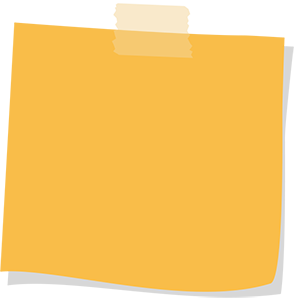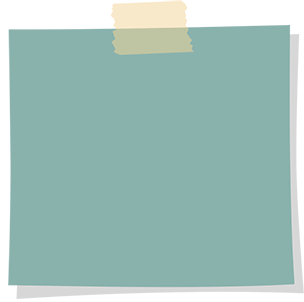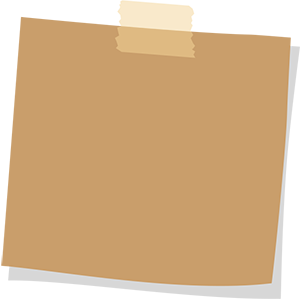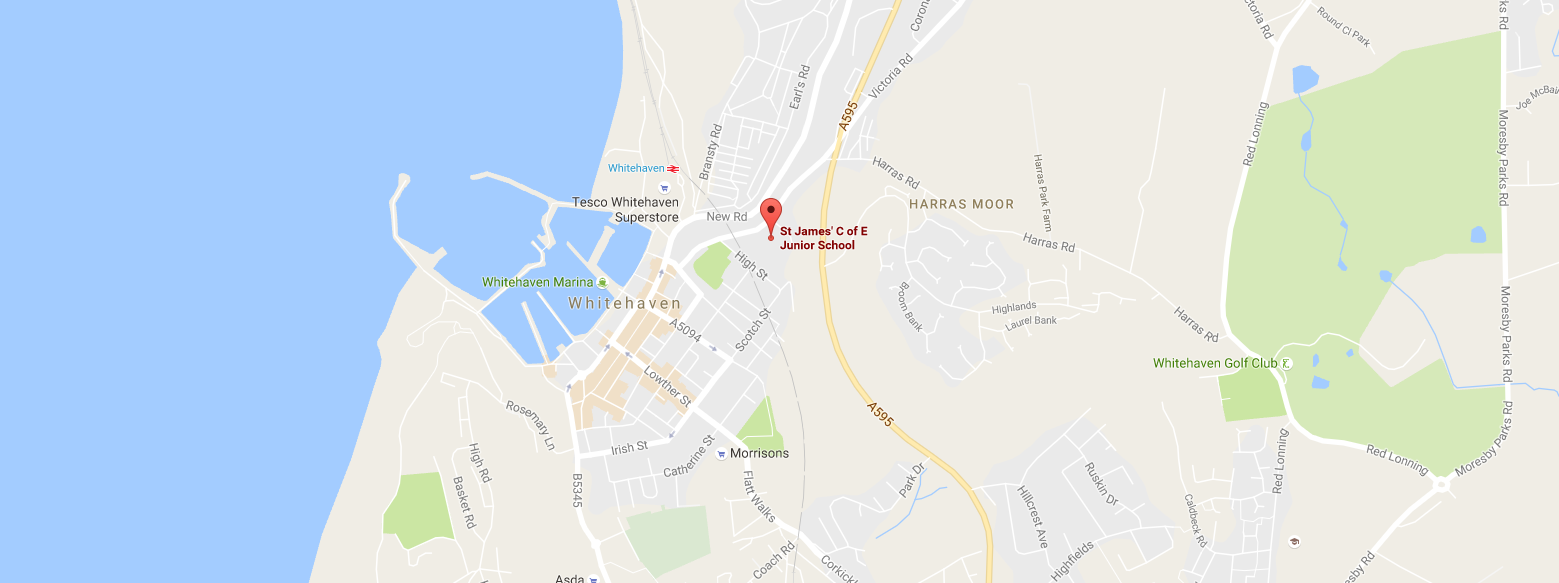Maths work in the hall

Lesson: Numeracy

Class: Mr Tyson - Class 2 Year: 2018 - 2019

Today we decided to get a bit more active in maths and use the space in the hall. The children answered a range of arithmetic questions before converting each answer into a letter using the conversion table they were provided with. The letters were then re-arranged to make a mathematical word - multiply.Attendance

Attendance week ending 21st June 2019 is 95.07%